# Variance

Calculates the empirical variance of its input signal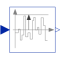# Information

This information is part of the Modelica Standard Library maintained by the Modelica Association.

This block calculates the empirical variance of its input signal. It is based on the formula (but implemented in a more reliable numerical way):

`y = mean(  (u - mean(u))^2  )`

The parameter t_eps is used to guard against division by zero (the variance computation starts at <simulation start time> + t_eps and before that time instant y = 0).

The variance of a signal is also equal to its mean power.

This block is demonstrated in the examples UniformNoiseProperties and NormalNoiseProperties.

# Parameters (1)

t_eps Value: 1e-7 Type: Time (s) Description: Variance calculation starts at startTime + t_eps

# Connectors (2)

u y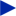Type: RealInput Description: Noisy input signal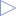Type: RealOutput Description: Variance of the input signal

# Used in Examples (2)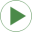UniformNoiseProperties Modelica.Blocks.Examples.NoiseExamples Demonstrates the computation of properties for uniformally distributed noiseNormalNoiseProperties Modelica.Blocks.Examples.NoiseExamples Demonstrates the computation of properties for normally distributed noise

# Used in Components (1)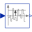StandardDeviation Modelica.Blocks.Math Calculates the empirical standard deviation of its input signal